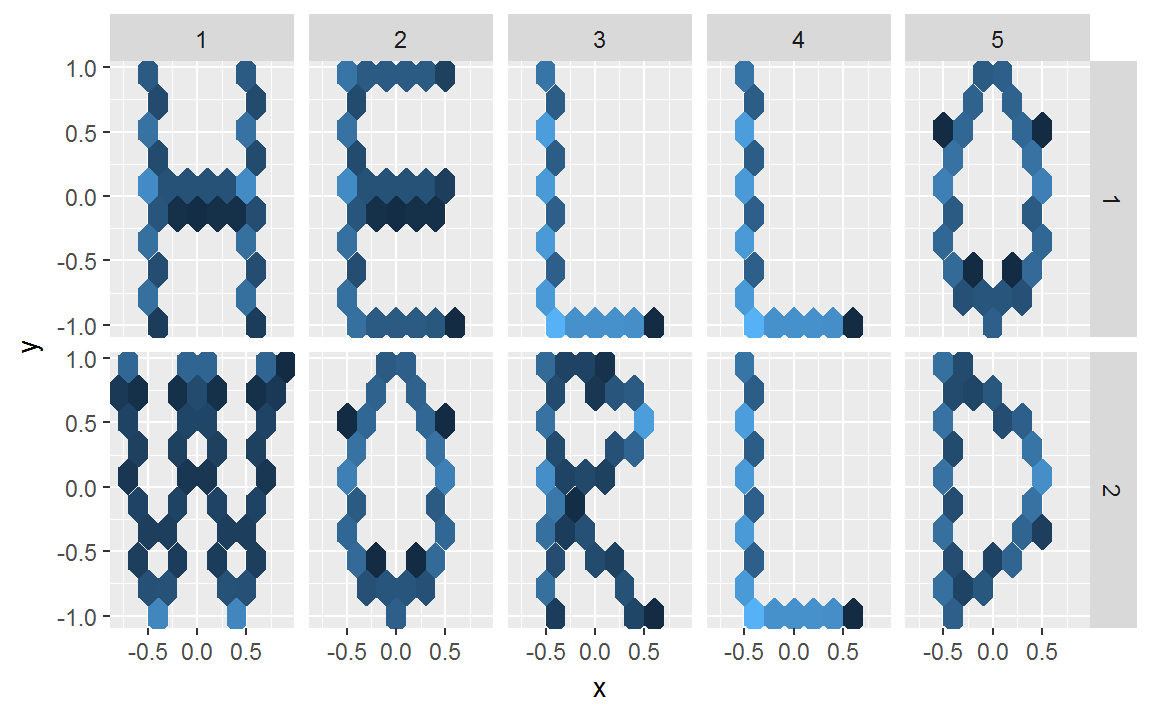## Tuesday, April 11, 2017

### Post 0

Welcome to my blog Bernhard Learns. My name is Bernhard Piskernik and I am a psychologist, data enthusiast, early career scientist, and father of two. My passion is learning new stuff and playing with data. I hope you join me on my journey through data science, psychometrics, and programming.

With this in mind

library(tidyverse)

Create data and plot…

## create letter coordinates

H <- data_frame(x = c(rep(-0.5, 200), seq(-0.5, 0.5, length.out = 100), rep(0.5,
200)), y = c(seq(-1, 1, length.out = 200), rep(0, 100), seq(-1, 1, length.out = 200)))

E <- data_frame(x = c(rep(-0.5, 200), rep(seq(-0.5, 0.5, length.out = 100),
3)), y = c(seq(-1, 1, length.out = 200), rep(-1:1, each = 100)))

L <- data_frame(x = c(rep(-0.5, 300), seq(-0.5, 0.5, length.out = 200)), y = c(seq(-1,
1, length.out = 300), rep(-1, each = 200)))

O <- data_frame(x = c(seq(-1, 1, length.out = 250)^2/2 - 0.5, seq(-1, 1, length.out = 250)^2/-2 +
0.5), y = rep(seq(-1, 1, length.out = 250), 2))

W <- data_frame(x = seq(-0.8, 0.8, length.out = 500), y = cos(seq(-2 * pi, 2 *
pi, length.out = 500)))

R <- data_frame(x = c(rep(-0.5, 200), seq(-1, 1, length.out = 200)^2 * -1 +
0.5, seq(-0.5, 0.5, length.out = 100)), y = c(seq(-1, 1, length.out = 200),
seq(1, 0, length.out = 200), seq(0, -1, length.out = 100)))

D <- data_frame(x = c(rep(-0.5, 200), seq(-1, 1, length.out = 300)^2 * -1 +
0.5), y = c(seq(-1, 1, length.out = 200), seq(-1, 1, length.out = 300)))

## build data_frame

hello_world <- bind_rows(H, E, L, L, O, W, O, R, L, D)

hello_world[["word"]] <- rep(1:2, each = 2500)
hello_world[["letter"]] <- rep(rep(1:5, each = 500), 2)

hello_world <- tbl_df(hello_world)

hello_world %>% mutate(word = factor(word), letter = factor(letter))

## create plot

h_plot <- hello_world %>% ggplot(aes(x = x, y = y)) + geom_hex(show.legend = F,
bins = 8) + facet_grid(word ~ letter)

Use plot as message for today:#### 1 comment:

1.Hello Bernhard.Welcome to the world of blogging. I am hoping to see your new post regarding your field anytime soon.Best of luck to you.

## Recommended Post

### Follow the white robot - Exploring retweets of Austrian politicians with Botometer in R

botometer_publish.utf8.md Hi folks! I guess you are aware that social medi...